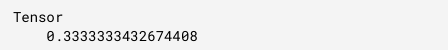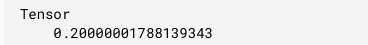# Tensorflow.js tf.losses.absoluteDifference() Function

Tensorflow.js is an open-source library developed by Google for running machine learning models and deep learning neural networks in the browser or node environment. It also helps the developers to develop ML models in JavaScript language and can use ML directly in the browser or in Node.js.

The Tensorflow.js tf.losses.absoluteDifference() function calculates the absolute difference loss between two given tensors.

Syntax:

```tf.losses.absoluteDifference(labels,
predictions, weights, reduction); ```

Parameters:

• labels: It specifies the truth output tensor. The absolute difference is predicted based on this tensor.
• predictions: It specifies the predicted output tensor with the same dimensions as labels.
• weights: It specifies a tensor of rank either equal to that of labels so that it can be broadcastable or 0. It is an optional parameter.
• reduction: It specifies the type of reduction to the loss. It is optional.

Return Value: It returns a tf.Tensor which is calculated by absoluteDifference() function.

Example 1: In this example we will take two 2d tensors as labels and prediction. Then we will find the absolute difference loss of these two.

## Javascript

 `// Importing the tensorflow.js library  ` `const tf = require(``"@tensorflow/tfjs"``);  ` ` `  `// Defining label tensor  ` `const y_true = tf.tensor2d([  ` `    ``[0., 1., 0.],   ` `    ``[0., 0., 0.]  ` `]);  ` ` `  `// Defining prediction tensor  ` `const y_pred = tf.tensor2d([  ` `    ``[1., 1., 0.],   ` `    ``[1., 0., 0 ]  ` `]);  ` ` `  `// Calculating absolute difference  ` `const absolute_difference =  ` `    ``tf.losses.absoluteDifference(y_true,y_pred)  ` `   `  `// Printing the output  ` ` ``absolute_difference.print()`

Output:Example 2: Taking weights of rank as of labels in the absolute function and then calculate the absolute difference.

## Javascript

 `// Importing the tensorflow.js library  ` `const tf = require(``"@tensorflow/tfjs"``);  ` `   `  `// Defining label tensor  ` `const y_true = tf.tensor2d(  ` `    ``[0., 1., 0., 0., 0., 0., 1.,   ` `    ``0., 1., 1., 0., 1.], [4, 3]  ` `);  ` `// Defining predicted tensor  ` `const y_pred = tf.tensor2d(  ` `    ``[1., 1., 0., 1., 0., 0., 1.,   ` `    ``1., 1., 0., 0., 1.], [4, 3]  ` `);  ` ` `  `   `  `// Calculating absolute difference ` `const absolute_difference = tf.losses.absoluteDifference(  ` `       ``y_true, y_pred, [0.7, 0.3, 0.2])  ` `absolute_difference.print() `

Output:Whether you're preparing for your first job interview or aiming to upskill in this ever-evolving tech landscape, GeeksforGeeks Courses are your key to success. We provide top-quality content at affordable prices, all geared towards accelerating your growth in a time-bound manner. Join the millions we've already empowered, and we're here to do the same for you. Don't miss out - check it out now!

Previous
Next